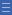## Featured resource

Home > Topdrawer > Mental computation > Big ideas > Key knowledge > Number facts: Addition and subtraction

# Number facts: Addition and subtraction

Ready recall of number facts is important for easing the load of working memory when more complex calculations are required. Number facts using addition and subtraction can help students to see patterns and relationships, particularly place value structures and the properties of operations.

Basic number facts are best developed through a process of noticing patterns and relationships.

For example, the development of subtraction facts draws on the inverse relationship of addition and subtraction.
To work out 15 – 7 = ? students might think 7 + ? = 15.

Some facts are more significant than others in terms of how easy they are to learn and their usefulness in calculation.

• Knowing doubles can assist in deriving near doubles such as 7 + 8.
• Facts to five are easily recognised and can be developed through imaging.

Combinations that add to 10 (e.g. 4 + 6 and 7 + 3) are essential for all addition strategies that use place value (e.g. up over 10 or back through 10 jumping).

Tens frames, cube stacks and finger patterns are all useful representations for learning these facts.

Watch the Using Tens Frames with Fingers video.

The bridging ten strategy is useful for facts such as 9 + 5.

Use of two tens frames provides an image of this strategy in action.

Students are more likely to remember facts if they link facts with others that they know.

Yes

Yes

Name Class SectionYear 3: Recall addition facts for single-digit numbers and related subtraction facts to develop increasingly efficient mental strategies for computa... Infobox 3Source Infobox 3